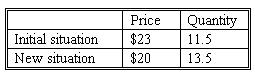### CFA Practice Question

There are 539 practice questions for this study session.

### CFA Practice Question

Compute the approximate elasticity of demand from the following data.A. 0.87
B. 1.14
C. 1.5
Explanation: Elasticity = [(11.5-13.5)/12.5]/[(23 -20)/21.5) = (2/12.5)/(3/21.5) = .16/.14 = 1.14

User Comment
PedroEdmundo Don't really agree with the explanation: I found 1.15. The formula is not about diving by the average bu the sum?
dimos ALWAYS put in the denominator the average
ljamieson and don't invert like i did ...
viannie hmmm ... I need to remember that elasticity is a measurement of % change of quantity due to % change in price. Per dollar change in price, how that affect the quantity! remembering the formula I found always put me in the spot of reversing the fraction and CFA always have both answer for options!
morek Remember it is absolute value. I always forget and think I am calculating incorrectly.
nneks don't care what they say the answer is 1.146667 which approximates to 1.15!
nneks shoot...I thought c was 1.15 not 1.5.....wow the little details thst we miss...
jpducros When dealing with elasticity calculation : 2 things to remember :
- always put quantities in the numerator.
- use the "average" in the denominator when calculating var quantities and var prices.
gill15 Remember guys. When we're doing the Arc convention or approximating its NOT the same as the percentage change of Q over Percentage change over P we usually use....it's a variation of that with the midpoint used instead of the initial value.